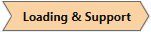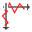Expert Modelling: Hands-on help from Rocscience Engineers. Register Here

# Seismic

1. Select the Loading & Supportworkflow tab.
2. Select Seismic Loadingfrom the Loading menu, or the right-click popup menu.
3. In the Seismic Load dialog, enter the Orientation and Seismic Load coefficient(s) as described below. Select OK.

## Orientation

There are three options for defining the Orientation of a pseudo-static Seismic Load:

• Slip Direction - the Slip Direction option allows you to input separate coefficients for Horizontal and Vertical Seismic Load. The Slip Direction option automatically applies the Horizontal seismic coefficient in the slip direction (i.e. direction of sliding). The Slip Direction is automatically calculated for each slip surface during the limit equilibrium analysis, and cannot be user defined. The Vertical seismic coefficient is applied in the vertical direction only and is independent of the Horizontal seismic load.
• Trend / Plunge - the Trend / Plunge option allows you to specify the 3D orientation of a seismic load by entering Trend and Plunge values. The Trend is measured in degrees clockwise from the north direction (north = the Y-axis of the model). Plunge is measured from the horizontal, with positive down convention. The seismic load coefficient is applied in the Trend/Plunge direction.
• Vector - the Vector option allows you to specify the 3D orientation of a seismic load in terms of XYZ components. The seismic load coefficient is applied in the Vector direction.

## Seismic Coefficient

The Seismic Coefficients are dimensionless coefficients which represent the (maximum) earthquake acceleration as a fraction of the acceleration due to gravity. Typical values are in the range of 0.1 to 0.3.

If seismic coefficients are defined, a seismic force will be applied to each column in the sliding mass as follows:

Seismic Force = Seismic Coefficient * Column Weight

= Seismic Coefficient * volume of column * Unit Weight of column material

The seismic force is applied through the geometric centroid of each column, according to the selected Orientation method as described above.

Note that the acceleration due to gravity (g = 9.81 m/s2 for metric units) is already incorporated into the material Unit Weight entered in the Define Material Properties dialog (the dimensions of Unit Weight are force / m3), and therefore "g" does not explicitly appear in the above equation.

## Excess Pore Pressure

The Excess Pore Pressure option in the Seismic Load dialog, will only be available if the Excess Pore Pressure option is selected in Project Settings > Groundwater > Advanced options.

If you select the Load creates excess pore pressure checkbox in the Seismic Load dialog, then a VERTICAL seismic load will generate excess pore pressure for all materials with B-bar > 0. This only applies to the VERTICAL component of seismic loads. It does NOT apply to the HORIZONTAL component of seismic loads. For more information see the Excess Pore Pressure topic.